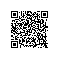# 【C#】3.算法温故而知新 - 快速排序using System;
using System.Collections.Generic;
using System.Linq;
using System.Text;

namespace ConsoleApplication1
{
public class Program
{
private static int[] a = new int { 6, 1, 2, 7, 9, 3, 4, 5, 10, 8 };//初始化一个数组,其中有10个数，这里假定是我们输入的数，需要从小到大排序
public static void Main(string[] args)
{
QuickSort(0, a.Length - 1);//最左边数和最右边数的下标
//输出排序后的结果
for (int i = 0; i < a.Length; i++)
Console.Write("  " + a[i]);
}

/// <summary>交换方法 </summary>
/// <param name="left">最左边数的下标</param>
/// <param name="right">最右边数的下标</param>
private static void QuickSort(int left, int right)
{
int i, j, t, temp;
if (left > right)//下标交错即返回
return;
temp = a[left];//temp中存的就是基准数,假定最左边的数为基准数
i = left;//左边数的下标
j = right;//右边数的下标

while (i != j)//当下标不相等时，直到i=j时停止
{
//顺序很重要，要先从右往左找
while (a[j] >= temp && i < j)//找到大于基准数的数
j--;
//再从从左往右找
while (a[i] <= temp && i < j)//找到小于基准数的数
i++;

//交换两个数在数组中的位置
if (i < j)
{
t = a[i];
a[i] = a[j];
a[j] = t;
}
}
//最终将基准数归位
a[left] = a[i];
a[i] = temp;

QuickSort(left, i - 1);//继续处理左边的，这里是一个递归的过程
QuickSort(i + 1, right);//继续处理右边的，这里是一个递归的过程
}

}
}使用钉钉扫一扫加入圈子
+ 订阅